# Let’s revisit √844

844 is repdigit 444 in BASE 14 because 4(14²) + 4(14¹) + 4(14⁰) = 844.

None of the following should surprise us:

• Since 844 is 444 in BASE 14, we know 4 is a factor of 844.
• (844)/2 = 422 which is 222 in BASE 14.
• (844)/4 = 211 which is 111 in BASE 14

Back when I wrote Post 266 I made some observations about the numbers 844, 845, 846, 847, and 848. Those are the smallest five consecutive numbers whose square roots can be simplified. Now that I’m writing Post 844, I am just as amazed by those five consecutive numbers and their square roots.Although I included that graphic and the next one in that previous post. Both of them are worth a second look.• 844 is a composite number.
• Prime factorization: 844 = 2 × 2 × 211, which can be written 844 = 2² × 211
• The exponents in the prime factorization are 2 and 1. Adding one to each and multiplying we get (2 + 1)(1 + 1) = 3 × 2  = 6. Therefore 844 has exactly 6 factors.
• Factors of 844: 1, 2, 4, 211, 422, 844
• Factor pairs: 844 = 1 × 844, 2 × 422, or 4 × 211
• Taking the factor pair with the largest square number factor, we get √844 = (√4)(√211) = 2√211 ≈ 29.051678# 411 Some Things You Should Know Before You Start a Blog

You’re passionate about some subject and are considering starting a blog. Here’s some things you should know first:

What your blog is about will eventually change to one degree or another. I have noticed many bloggers redefine themselves, some more than others. My blog was initially a way to share a factoring puzzle I invented, but most of the people who visit my blog are not looking for puzzles; they want to find the factors of a particular number. After all, my blog name implies that factors can be found here. To satisfy these viewers, I now give the factoring information for the number 411:

• 411 is a composite number.
• Prime factorization: 411 = 3 x 137
• The exponents in the prime factorization are 1 and 1. Adding one to each and multiplying we get (1 + 1)(1 + 1) = 2 x 2 = 4. Therefore 411 has exactly 4 factors.
• Factors of 411: 1, 3, 137, 411
• Factor pairs: 411 = 1 x 411 or 3 x 137
• 411 has no square factors that allow its square root to be simplified. √411 ≈ 20.2731When I first noticed that people were visiting my blog because they wanted to know the factors of a number, I resisted changing, but later I gave in. I even edited all my previous blog posts to include factored numbers. Eventually I added the square roots of numbers and indicated if those square roots could be simplified. Still I keep my original intent and publish the puzzles I create.

Coming up with imaginative titles for EVERY blog post can be tedious or even impossible. Many people judge whether or not a post is worth reading by the title. Sometimes a great title pops into my head, but most of the time I’ve got nothing. I started giving my puzzle posts boring titles like “Level 3”  and actually had several posts with that same name. When my stats page told me that someone liked or visited a post called Level 2, I wasn’t sure which post that was. When I started factoring numbers, it solved my problem of giving each post a distinctive title even if it was still a boring name. Now I often use unimaginative titles like “406 and Level 4”.

Blogging uses up more time than you imagine it will. I once read a very long, hilariously funny, grammatically perfect post that the author claimed he wrote during his lunch time. I find that claim very hard to believe because it certainly doesn’t work that way for me. I can spend 15-30 minutes reading or writing blogs, but the clock at my house indicates that I actually spent two hours or more. No matter what clock I use, it’s the same story.

Most bloggers don’t make money blogging. If you want to make money on WordPress, you have to have enough views, you have to purchase your own domain name, and you have to sign up for WordAds. After some consideration, I took this step in August 2014. I registered my domain name for \$18 a year and signed up for WordAds. I also pay another \$8 each year to keep my contact information private.

How many views do you have to have to make money on WordPress? Update: Since writing this post, WordPress allows ALL of its bloggers to sign up for Wordads. Now every blog with a registered domain name is a potential money maker, even if it turns out to be just a little bit of money each month. The amount of money that you’ll make will depend on how many views you have and where those views come from.

Was it worth it to register your own domain name? Yes, the number of views I’ve received after registering is much, much greater than it was before I registered. It is much easier to tell friends and acquaintances to look for Findthefactors.com than it was to tell them that name with wordpress added to it. Even though it cost more money than I might have made back, it helps me reach more viewers, and that helps me meet my original objective. The only drawback is that if don’t renew my registration every year Findthefactors.com could become what they call a parking lot. I would have no control over what people would see if they went to the site. If you read the fine print when you register your domain, this stipulation is made quite clear. Somebody else could put whatever they want on the unregistered site, including adult content. I intend to continue registering Findthefactor.com for the rest of my life. Hopefully my children and grandchildren will also after I’m gone.

If you’re still thinking of starting a blog, do it because you love a particular subject. Do it because it pleases you. There will be days when absolutely nobody else looks at your blog. You will gain some followers, but some of those followers will get discouraged with their statistics and quit blogging and quit reading your blog. You will also get some followers who follow you for the sole purpose of trying to get you to follow them back. Once you follow them, they never look at your blog again. If you blog, you have to do it for yourself, and if anyone else enjoys it, that is a bonus.

I chose WordPress because it allows me to put excel documents on my blog. (Blogspot didn’t.) Unfortunately people who view my blog on their smart phones can’t see those documents. I hope there are a few teachers out there who at least occasionally look at my blog on their home or school computers and print out the puzzles for their classes. That would make my decision to add those documents definitely worth it.

Fellow bloggers, what other things do you think new bloggers should know?

# 40% of Numbers Up To 400 Have Square Roots That Can Be Simplified

• 400 is a composite number.
• Prime factorization: 400 = 2 x 2 x 2 x 2 x 5 x 5, which can be written 400 = (2^4) x (5^2)
• The exponents in the prime factorization are 4 and 2. Adding one to each and multiplying we get (4 + 1)(2 + 1) = 5 x 3 = 15. Therefore 400 has exactly 15 factors.
• Factors of 400: 1, 2, 4, 5, 8, 10, 16, 20, 25, 40, 50, 80, 100, 200, 400
• Factor pairs: 400 = 1 x 400, 2 x 200, 4 x 100, 5 x 80, 8 x 50, 10 x 40, 16 x 25, or 20 x 20
• 400 is a perfect square. √400 = 20A few months ago I made a chart showing the number of factors for the first 300 counting numbers. Since this is my 400th post, I’d like to include a chart showing the number of factors for all the numbers from 301 to 400. I’m also interested in consecutive numbers with the same number of factors and whether or not the square root of a number can be reduced. The red numbers have square roots that can be reduced.The longest streak of consecutive numbers with the same number of factors is only three. There are three sets of three consecutive numbers on this chart. (Between 200 and 300 there was a streak of four consecutive numbers with six factors each.)

How do the number of factors of these 100 numbers stack up against the previous 300? The following chart shows the number of integers with a specific number of factors and how many of those integers have reducible square roots: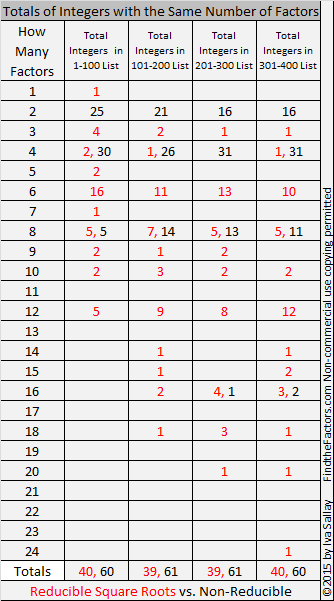• 39.5% or slightly less than 40% of the numbers up to 400 have reducible square roots.
• Most of these numbers have 2, 4, or 8 factors. Numbers with two factors are prime numbers. Almost all numbers with four factors are the product of two different prime numbers, and nearly two-thirds of the numbers with eight factors are the product of three different prime numbers.
• There isn’t much change between the percentages of reducible square roots from one list to the next.

# 360 What Can You Do With Fraction Circles?

360 has more factors than any previous number. 240 and 336 held the previous record of 20 factors for each of them. How many factors do you think 360 has? Scroll down to the end of the post to find out.

360 can be evenly divided by every number from one to ten except seven, so it was a good number for the ancients to choose when they divided the circle into 360 degrees.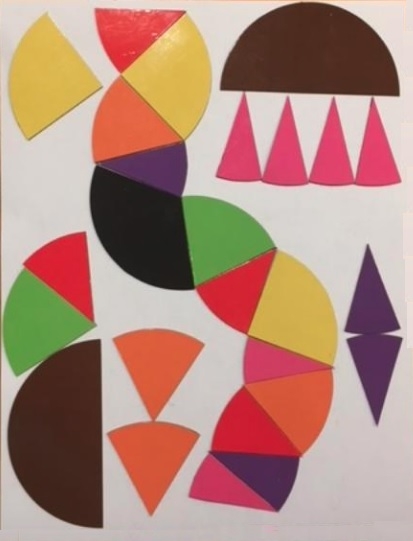I bought a few fraction circles. Each 51 piece set consists of 1 whole circle as well as circles divided into 2 halves, 3 thirds, 4 fourths, 5 fifths, 6 sixths, 8 eighths, 10 tenths, and 12 twelves. What can you do with fraction circles? You can do a lot with them no matter what your age.

Art and Mathematics

The fraction circle shapes can be used just as tangram shapes to create artwork, big or small. A couple of cool symmetric designs can be found at fraction-art and fraction-circle-art. Adding rectangular fraction pieces will increase the possibilities. Here are some simple artistic designs.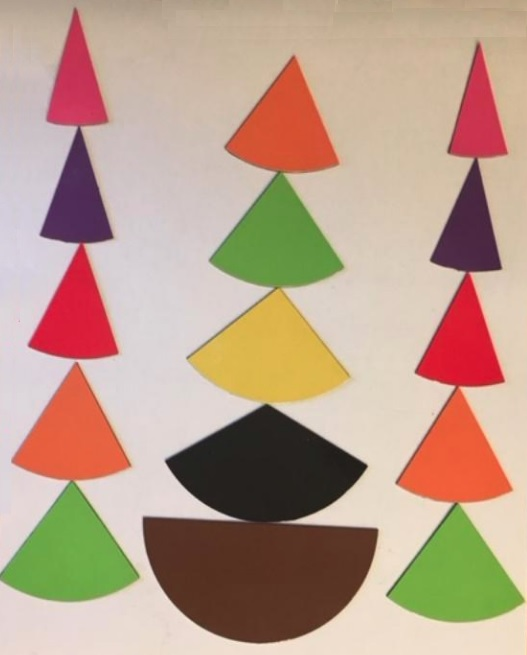Fraction Relationships

You can use fraction circle shapes to explore the relationship between fractions such as ½, ¼, and  ⅟₈;  ⅟₃, ⅟₆  and ⅟₁₂; or ½, ⅟₅ and ⅟₁₀: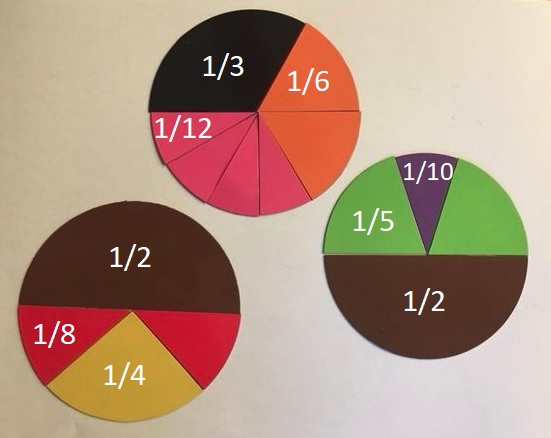Areas of Parallelograms, Trapezoids, and CirclesThe picture above shows what happens when the circle is divided into four, six, eight, ten or twelve equal wedges, and the wedges are arranged into something that resembles a parallelogram. This idea can be so easily duplicated with these fraction circles without any cutting.

Here are some good questions to ask:

1. What happens to the top and bottom of the shape when the number of wedges increases?
2. Sometimes the resulting shape will look like a trapezoid, and sometimes it looks more like a parallelogram. Why does that happen?We know that the circumference of any circle is 2πr with π defined as the circumference divided by the radius. π is the same value no matter how big or small the circle is.

We can calculate the area of any of the parallelogram-like shapes or trapezoid-like shapes above. Let’s call the length of the bottom of the shape b₁ and the length of the top b₂. The area of the resulting shape is calculated: A = ½ · (b₁ + b₂) · h. Since b₁ + b₂ = 2πr, and the height equals the radius, we can write our formula for the area of a circle as A = ½ · 2πr · r = πr².

This exercise demonstrates that the area of rectangles, parallelograms, trapezoids, and circles are all related!

Introduction to Pie Charts

Pie charts are a great way to display data when we want to look at percentages of a whole. If you use fraction circles, you are limited to using only to certain percentages, but they can still make a good introduction to the subject. To make the pie chart work either the total of all the degrees will have to equal 360 or the total of all the percents will have to equal 100:After a brief introduction using the fraction circles, try Kids Zone Create a Graph. It’s really easy to use!

Exploring Perimeter and Introducing Radians in Trigonometry

The perimeter of each fraction circle piece can be calculated. If the r = 1, the circumference of the circle is 2π, and we can see an important relationship between the degrees and the perimeter of each piece.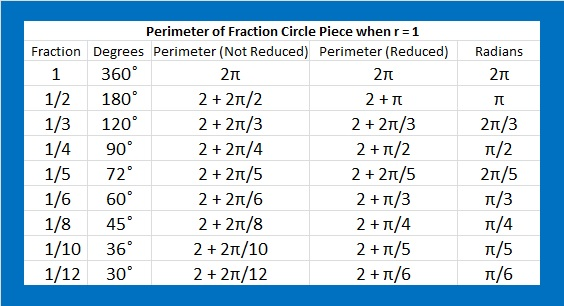What experiences have YOU had with circle fractions? Did you find them frustrating or enlightening? Personally, I like them very much, but I wish they had also been cut into ninths.

Here are some facts about the number 360:

The interior angles of every convex or concave quadrilateral total 360 degrees.

The exterior angles of every convex or concave polygon also total 360 degrees.

Here is all the factoring information about 360:

• 360 is a composite number.
• Prime factorization: 360 = 2 x 2 x 2 x 3 x 3 x 5, which can be written 360 = 2³·3²·5
• The exponents in the prime factorization are 3, 2 and 1. Adding one to each and multiplying we get (3 + 1)(2 + 1)(1 + 1) = 4 x 3 x 2 = 24. Therefore 360 has exactly 24 factors.
• Factors of 360: 1, 2, 3, 4, 5, 6, 8, 9, 10, 12, 15, 18, 20, 24, 30, 36, 40, 45, 60, 72, 90, 120, 180, 360
• Factor pairs: 360 = 1 x 360, 2 x 180, 3 x 120, 4 x 90, 5 x 72, 6 x 60, 8 x 45, 9 x 40, 10 x 36, 12 x 30, 15 x 24 or 18 x 20
• Taking the factor pair with the largest square number factor, we get √360 = (√10)(√36) = 6√10 ≈ 18.974# 269 and Five More Consecutive Square Roots

• 269 is a prime number.
• Prime factorization: 269 is prime.
• The exponent of prime number 269 is 1. Adding 1 to that exponent we get (1 + 1) = 2. Therefore 269 has exactly 2 factors.
• Factors of 269: 1, 269
• Factor pairs: 269 = 1 x 269
• 269 has no square factors that allow its square root to be simplified. √269 ≈ 16.401How do we know that 269 is a prime number? If 269 were not a prime number, then it would be divisible by at least one prime number less than or equal to √269 ≈ 16.401. Since 269 cannot be divided evenly by 2, 3, 5, 7, 11, or 13, we know that 269 is a prime number.

As I have previously written, 844, 845, 846, 847, and 848 are the smallest FIVE consecutive numbers whose square roots can be simplified. Here are the second smallest FIVE with the same property.The first number in the second set, 1680, equals 2 x 840 which is very close to the first number in the first set. Will strings of five consecutive numbers with reducible square roots occur about once every 850 numbers?

We can find the number of factors for these numbers by examining their prime factorizations.The number of factors for each of the integers in this second set ranges from 3 to 40. Only two of the integers have the same number of factors. Finding another string of four or more numbers that have reducible square roots as well as the same number of factors may be difficult.

# 266 Why 8 Consecutive Numbers with 6 Factors is Impossible

• 266 is a composite number.
• Prime factorization: 266 = 2 x 7 x 19
• The exponents in the prime factorization are 1, 1, and 1. Adding one to each and multiplying we get (1 + 1)(1 + 1)(1 + 1) = 2 x 2 x 2 = 8. Therefore 266 has 8 factors.
• Factors of 266: 1, 2, 7, 14, 19, 38, 133, 266
• Factor pairs: 265 = 1 x 266, 2 x 133, 7 x 38, or 14 x 19
• 266 has no square factors that allow its square root to be simplified. √266 ≈ 16.3095242, 243, 244, and 245 are the smallest four consecutive numbers that have the same number of factors. Each of them has exactly six factors, and as a result each one of their square roots can be simplified. Is it possible to have a longer string of consecutive numbers with exactly six factors? I don’t know yet. It seems reasonable that it could happen, so I am on the lookout for five, six, or seven consecutive numbers that have exactly six factors.

I won’t bother looking for a string of eight or more numbers with six factors. I already know that would be impossible. Here’s an example illustrating why:846 ruins the run because it has an additional prime factor that doubles the number of factors that 846 has in all. Further down the number line that problem might be overcome in a different set of consecutive numbers.

However, the problem with 848 is a recurring problem that will never be overcome. 848 is divisible by 8, as is every eighth number. The prime factorization of numbers that are divisible by eight must contain a power of two that is greater than or equal to three. Its number of factors calculation would have to be at least (3 + 1)(1 + 1) = 4 x 2 = 8. (The ONLY number divisible by 8 that has exactly 6 factors is 32.)

Even though the numbers from 844 to 848 don’t have the same number of factors, they still have a distinction. They are the smallest five consecutive numbers whose square roots can be simplified!# A Forest of 240 Factor Trees

### Factor Trees for 240:

Because 240 has so many factors, it is possible to make MANY different factor trees that create a forest of 240 factor trees. This post only contains eleven of those many possibilities. The two trees below demonstrate different permutations that can be made from the same basic tree. The mirror images of both, as well as mirror images of parts of either tree, would be other permutations.A good way to make a factor tree for a composite number is to begin with one of its factor pairs and then make factor trees for the composite numbers in that factor pair.

This forest of 240 factor trees is dedicated to Joseph Nebus. Read the comments to his post, You might also like, because, I don’t know why, to discover why I was inspired to create images of parts of this forest.

### Factors of 240:

• 240 is a composite number.
• Prime factorization: 240 = 2 x 2 x 2 x 2 x 3 x 5, which can be written 2⁴ x 3 x 5
• The exponents in the prime factorization are 4, 1 and 1. Adding one to each and multiplying we get (4 + 1)(1 + 1)(1 + 1) = 5 x 2 x 2 = 20. Therefore 240 has 20 factors.
• Factors of 240: 1, 2, 3, 4, 5, 6, 8, 10, 12, 15, 16, 20, 24, 30, 40, 48, 60, 80, 120, 240
• Factor pairs: 240 = 1 x 240, 2 x 120, 3 x 80, 4 x 60, 5 x 48, 6 x 40, 8 x 30, 10 x 24, 12 x 20, or 15 x 16
• Taking the factor pair with the largest square number factor, we get √240 = (√16)(√15) = 4√15 ≈ 15.492### Sum-Difference Puzzle:

60 has six factor pairs. One of those factor pairs adds up to 17, and a different one subtracts to 17. Can you find those factor pairs to solve the first puzzle below?

240 has ten factor pairs. One of them adds up to 34, and another one subtracts to 34. If you can identify those factor pairs, then you can solve the second puzzle.The second puzzle is really just the first puzzle in disguise. Why would I say that?

### Another Fact about the Number 240:

2 + 4 + 6 + 8 + 10 + 12 + 14 + 16 + 18 + 20 + 22 + 24 + 26 + 28 + 30 = 240; that’s the sum of the first 15 even numbers.

# 55 and Square Roots for Third Grade

55 is a composite number. 55 = 1 x 55 or 5 x 11. Factors of 55: 1, 5, 11, 55. Prime factorization: 55 = 5 x 11.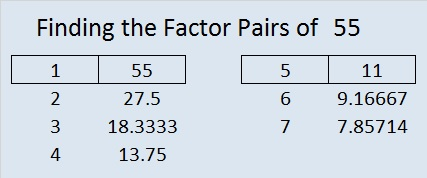Sometimes 55 is a clue in the FIND THE FACTORS 1 – 12 puzzles. Even though it has other factors, the puzzle only uses 5 x 11 = 55.

——————————————————————————————-

What is the square root of a number? If you had a square made with 9 squares, how many squares would be on each side?Even a third grader can count 3 squares on each side. That is one way to explain why √9 = 3 to someone so young. Here’s another explanation:

1. 1 x 1 = 1, so √1 = 1
2. 2 x 2 = 4, so √4 = 2
3. 3 x 3 = 9, so √9 = 3
4. 4 x 4 = 16, so √16 = 4
5. 5 x 5 = 25, so √25 = 5
6. 6 x 6 = 36, so √36 = 6
7. 7 x 7 = 49, so √49 = 7
8. 8 x 8 = 64, so √64 = 8
9. 9 x 9 = 81, so √81 = 9
10. 10 x 10 = 100, so √100 = 10

These are the kinds of square roots that third grade students may need to know. When they get older they will learn about other square roots like √2 ≈ ±1.414, but third graders don’t need to worry about those yet.

On January 24 someone hurriedly typed “aquare roots for third grade” into a search engine, and a post from this blog came up. The person took ONE quick look and closed the post and the blog just as hurriedly. I’m not exactly sure what aquare or square roots for third grade means, but I felt bad that the blog disappointed this unknown person. I thought, “That person MAY have been searching for something that actually might be on the blog SOMEWHERE…..Perhaps a puzzle with perfect square clues would have been a welcome sight.” Hey, I can dream, can’t I?

To solve today’s puzzle (It actually has perfect square clues!), you will have to take the square roots of the clues, and place those square roots in the top row as well as the first column. After you write down all those square roots, figure out what numbers go in the empty cells so that the entire puzzle works as a multiplication table.These square roots may or may not be the square roots for third grade that person was looking for. Nevertheless, I have personally seen several bright THIRD grade students solve similar perfect square puzzles that I’ve made. This week’s puzzles are available in an excel file here. If you have a spreadsheet program on your computer, you can access it. If you enable editing in excel, you can type your answers directly onto the puzzle, and you can also easily print the puzzles. Here are the factors for last week’s level 1 puzzle: